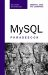MySQL Table Terminology

 MySQL Table TerminologyThe components of a MySQL table are A table is a structured collection of data, which is composed of...a body, that contains rows of data and...a table definition that contains columns that describe the type of data contained in the rows.Each column has a name (such as book_id) and a type (such as INTEGER). Each type is a defined set of values (like integers or letters in the alphabet). If data is of a given type, it must be one of the values from that type (for example, a column with type INTEGER must contain a whole number value such as 1 or 6502, not a string value such as one or a decimal number such as 3.14).Each row contains one value for every column in the heading. The values must be of the type defined in the column to which they correspond. The column book_id is of type SMALLINTonly values of that type may be stored in that position in the row.The number of columns a relation has defines its degree.The number of rows a relation has defines its cardinality.MySQL Phrasebook
ISBN: 0672328399
EAN: 2147483647
Year: 2003
Pages: 130

Similar book on Amazon